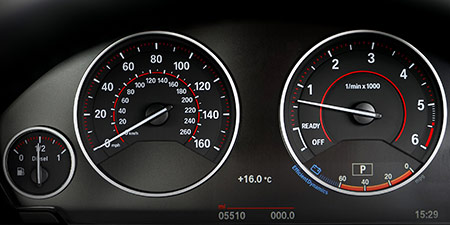# Speed of sound to Kilometers per second (km/s)

Thema Units of speed ﹣ Converter Speed of sound to Kilometers per second

Here you can convert the Speed unit Speed of sound into the unit Kilometers per second and vice versa you can convert Kilometers per second into Speed of sound. By clicking the "Swap units" icon, you will always obtain the desired conversion in the calculation result. With the following calculator you can also calculate any other Speed unit.

## Info about "Speed of sound"

Definition: If an object travels at the speed of sound in air at 20 degrees Celsius for one second, it travels a distance of 343 meters per second. Actually, it is equivalent to 1.234,8 km/h.

The speed of sound is often illustrated by an example of a thunderstorm: if lightning strikes within 3 seconds of the thunder sound, then the thunderstorm is about 1 kilometer away. Another example, in a 100-meter sprint, the starting shot is fired directly next to the starting blocks. Imagine, if the start shot was fired at the finish line, it would take about 0.3 seconds of time to hear the shot in the start blocks.

## Info about "Kilometers per second"

Kilometer per second is part of the metric system and it is derived from the standard unit of speed, meter per second (m/s). This is because both meters and seconds are defined as the basic units for length and time respectively.

This unit is defined as follows: An object moving at "1 km/s" per second will cover one kilometer. "km/s" is approved to use in the international system of units (SI).

## Formula for the conversion of Speed of sound to Kilometers per second (km/s) and vice versa

The calculation from Speed of sound to Kilometers per second shall be made using the following conversion formula:

Conversion formula Speed of sound to Kilometers per second

Determine the number of Kilometers per second from Speed of sound

Speed of sound × 0.343

## Formula for the conversion of Kilometers per second (km/s) to Speed of sound

The calculation from Kilometers per second to Speed of sound shall be made using the following conversion formula:

Conversion formula Kilometers per second to Speed of sound

Determine the number of Speed of sound from Kilometers per second

Kilometers per second × 2.9154518950437

## Overview table : How many Speed of sound are in Kilometers per second ?

Speed of sound ⇒ Kilometers per second km/s
0.01   are  0.00343 km/s
0.02   are  0.00686 km/s
0.03   are  0.01029 km/s
0.04   are  0.01372 km/s
0.05   are  0.01715 km/s
0.06   are  0.02058 km/s
0.07   are  0.02401 km/s
0.08   are  0.02744 km/s
0.09   are  0.03087 km/s
0.10   are  0.03430 km/s
0.20   are  0.06860 km/s
0.30   are  0.10290 km/s
0.40   are  0.13720 km/s
0.50   are  0.17150 km/s
0.60   are  0.20580 km/s
0.70   are  0.24010 km/s
0.80   are  0.27440 km/s
0.90   are  0.30870 km/s
1   corresponds to  0.34300 km/s
2   are  0.68600 km/s
3   are  1.02900 km/s
4   are  1.37200 km/s
5   are  1.71500 km/s
6   are  2.05800 km/s
7   are  2.40100 km/s
8   are  2.74400 km/s
9   are  3.08700 km/s
10   are  3.43000 km/s
20   are  6.86000 km/s
30   are  10.29000 km/s
40   are  13.72000 km/s
50   are  17.15000 km/s
60   are  20.58000 km/s
70   are  24.01000 km/s
80   are  27.44000 km/s
90   are  30.87000 km/s
100   are  34.30000 km/s
200   are  68.60000 km/s
300   are  102.90000 km/s
400   are  137.20000 km/s
500   are  171.50000 km/s
600   are  205.80000 km/s
700   are  240.10000 km/s
800   are  274.40000 km/s
900   are  308.70000 km/s
1 000   are  343 km/s

## Overview table : How many Kilometers per second are in Speed of sound ?

Kilometers per second km/s ⇒ Speed of sound
0.01 km/s  are  0.02915
0.02 km/s  are  0.05830
0.03 km/s  are  0.08746
0.04 km/s  are  0.11661
0.05 km/s  are  0.14577
0.06 km/s  are  0.17492
0.07 km/s  are  0.20408
0.08 km/s  are  0.23323
0.09 km/s  are  0.26239
0.10 km/s  are  0.29154
0.20 km/s  are  0.58309
0.30 km/s  are  0.87463
0.40 km/s  are  1.16618
0.50 km/s  are  1.45772
0.60 km/s  are  1.74927
0.70 km/s  are  2.04081
0.80 km/s  are  2.33236
0.90 km/s  are  2.62390
1 km/s  corresponds to  2.91545
2 km/s  are  5.83090
3 km/s  are  8.74635
4 km/s  are  11.66180
5 km/s  are  14.57725
6 km/s  are  17.49271
7 km/s  are  20.40816
8 km/s  are  23.32361
9 km/s  are  26.23906
10 km/s  are  29.15451
20 km/s  are  58.30903
30 km/s  are  87.46355
40 km/s  are  116.61807
50 km/s  are  145.77259
60 km/s  are  174.92711
70 km/s  are  204.08163
80 km/s  are  233.23615
90 km/s  are  262.39067
100 km/s  are  291.54519
200 km/s  are  583.09037
300 km/s  are  874.63556
400 km/s  are  1 166.18075
500 km/s  are  1 457.72594
600 km/s  are  1 749.27113
700 km/s  are  2 040.81632
800 km/s  are  2 332.36151
900 km/s  are  2 623.90670
1 000 km/s  are  2 915.45189

## Source information

As source for the information in the "Units of speed" category, we have used in particular:

## Last update on May 3, 2022

The pages of the "Units of speed" category were last editorially reviewed by Stefan Banse on May 3, 2022. They all correspond to the current status.

### Previous changes on October 9, 2021

• November 9, 2020: Publication of the Speed converter
• Editorial revision of all texts in this category